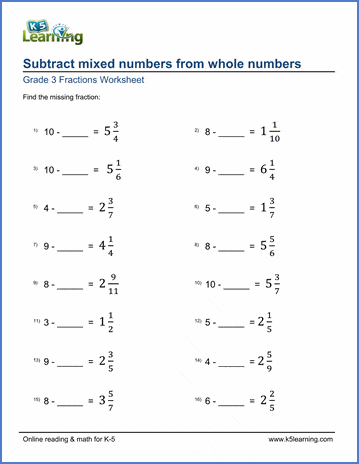# Subtract mixed numbers from whole numbers

## Fraction worksheets: subtracting mixed numbers from whole numbers

Below are six versions of our grade 3 math worksheet on subtracting mixed numbers from whole numbers; students must find the missing number from the subtraction equation.  Answers are given as mixed numbers. These worksheets are pdf files.## More fractions worksheets

Explore all of our fractions worksheets, from dividing shapes into "equal parts" to multiplying and dividing improper fractions and mixed numbers.

## What is K5?

K5 Learning offers reading and math worksheets, workbooks and an online reading and math program for kids in kindergarten to grade 5.  We help your children build good study habits and excel in school.